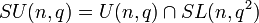# Special unitary group

## Definition

Suppose$L$ is a separable quadratic extension of a field$K$ and$\sigma:L \to L$ is the unique automorphism of$L$ that fixes$K$ pointwise. The special unitary group of degree$n$ for this quadratic extension, denoted$SU(n,L)$ (if the extension being referred to is understood) is defined as the subgroup of the special linear group$SL(n,L)$ comprising those matrices on which the transpose-inverse map gives the same result as the entry-wise application of$\sigma$.$SU(n,L) = \{ A \in SL(n,L) \mid \sigma(A) = (A^t)^{-1} \}$

Here,$\sigma(A)$ is the matrix obtained by applying$\sigma$ to each of the entries of$A$.

Alternatively, we can define it as the intersection of the unitary group and the special linear group, both viewed as subgroups of the general linear group:$SU(n,L) = U(n,L) \cap SL(n,L)$

### For the real and complex numbers

The most typical usage of the term special unitary group is in the context where$K$ is the field of real numbers,$L$ is the field of complex numbers, and the automorphism$\sigma$ is complex conjugation. In this case, the group$SU(n,\mathbb{C})$ is the subgroup of the special linear group$SL(n,\mathbb{C})$ comprising those matrices whose complex conjugate equals the transpose-inverse. When it's understood that we are working over the complex numbers, this group is sometimes just denoted$SU(n)$.

### For a finite field

If$K$ is the (unique up to isomorphism) finite field of size a prime power$q$, there is a unique quadratic extension$L$ of$K$, and this extension is separable. The extension field is the finite field (unique up to isomorphism) of order$q^2$. The automorphism$\sigma$ is the map$x \mapsto x^q$. The special unitary group for this extension may be denoted$SU(n,q)$ (the more standard choice) or$SU(n,q^2)$ (a less standard choice). Note that due to the ambiguity of notation, it is important to understand from context what exactly is meant.

Note that, if we denote this group by$SU(n,q)$, then, somewhat confusingly:$SU(n,q) = U(n,q) \cap SL(n,q^2)$